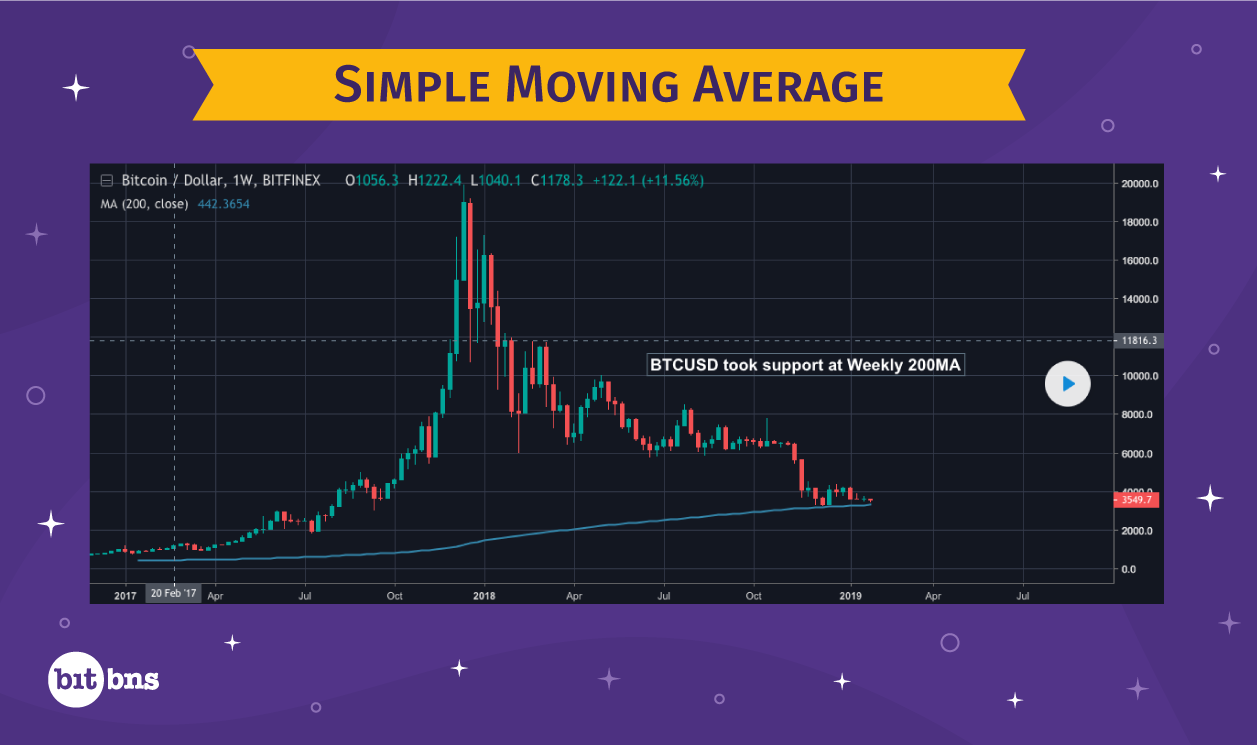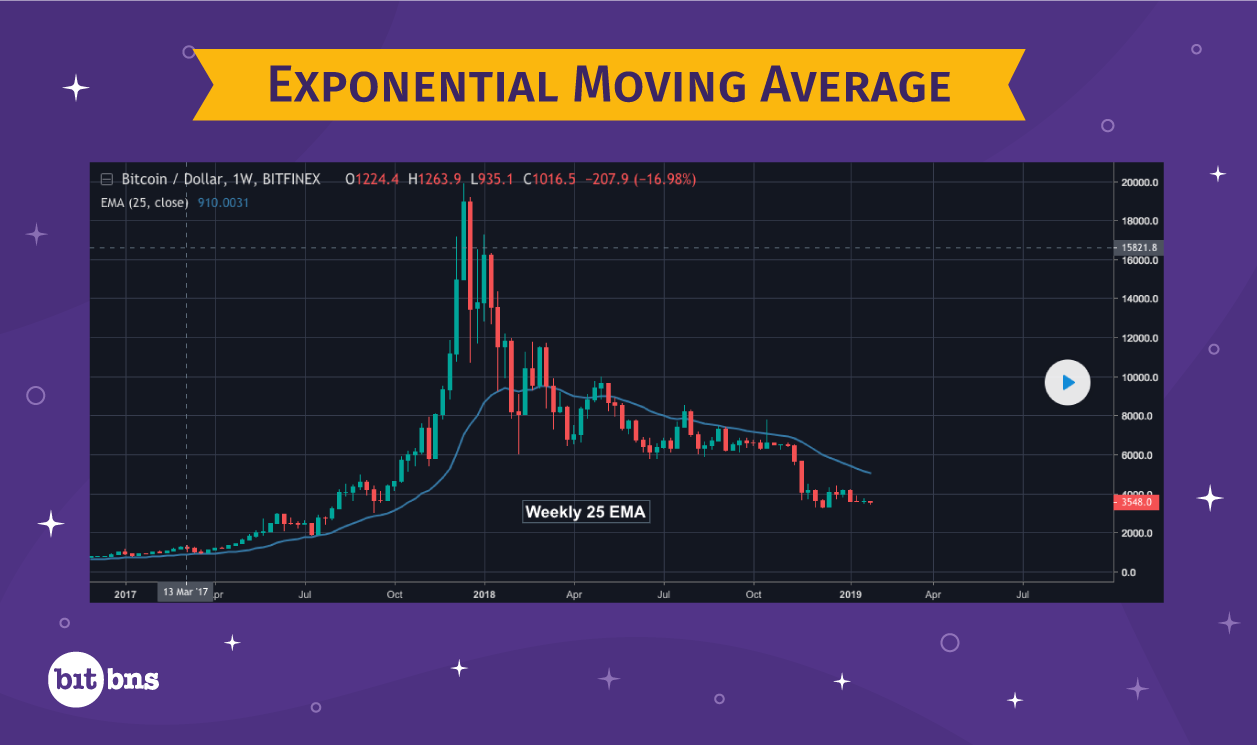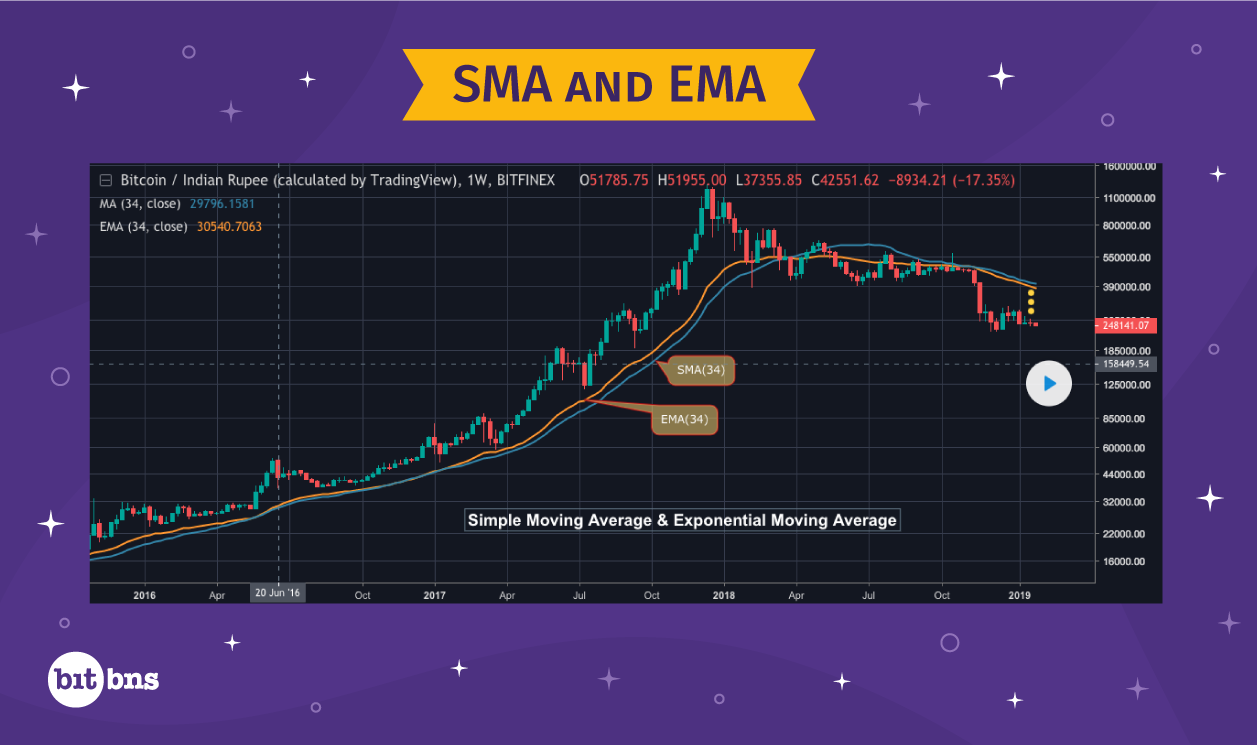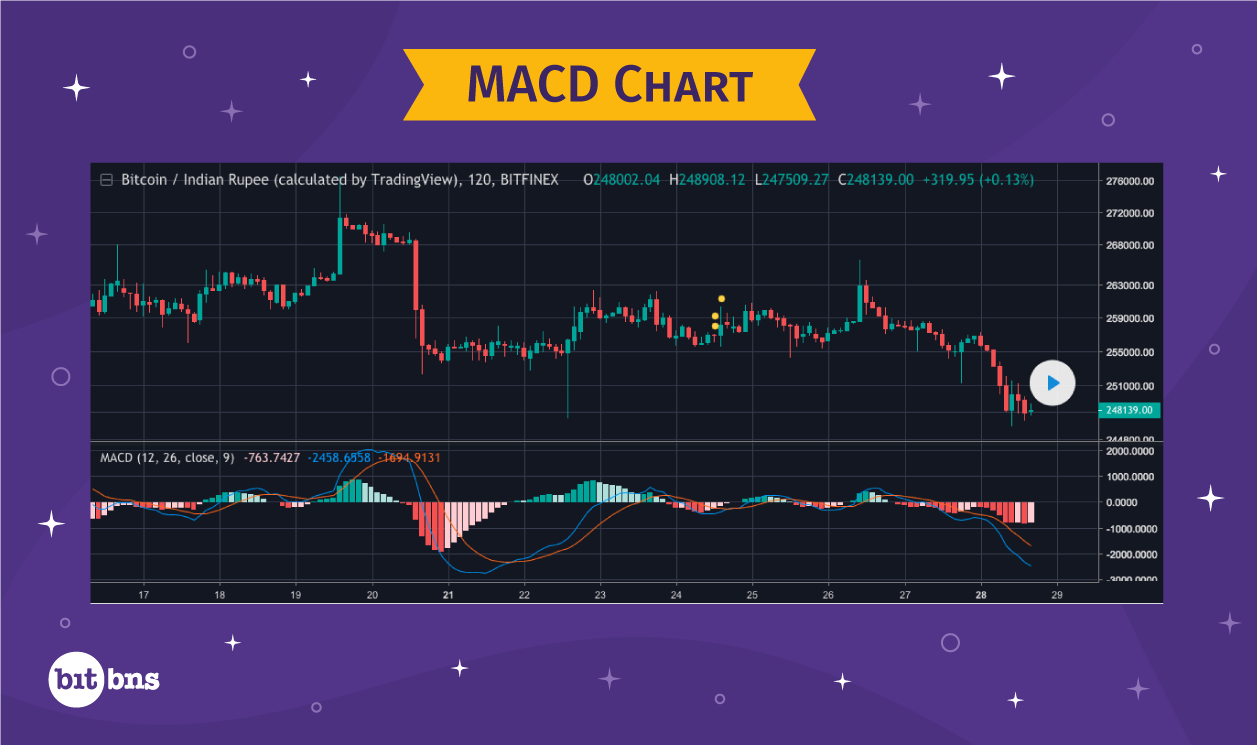# Moving AveragesThe most simplistic way to define moving averages is an average value that keeps moving or changing by addition or subtraction of data. To understand moving averages, you must first learn calculating average for a group of numbers.
How does one calculate the average of 5 numbers? Let’s see.
Let us assume there are five numbers for which we need to calculate average: (4, 7, 3, 6, 9)
To calculate the average or mean, we add these five numbers and divide the sum by 5.

So, Average: (4+7+3+6+9)/5 = 5.8.

Now I’m going to make a small change here. I am going to remove the first number in the series (4, 7, 3, 6, 9), introduce a new number every time I remove the first number in the series, and calculate the new average.
For example –
I am going to remove 4 and introduce 10 to the series.
The new average – (7+3+6+9+10)/5 = 7

Once again, I am going to remove the first number, which is 7, in the new series, and introduce 2.
The new average – (3+6+9+10+2)/5 = 6

Again, I’ll remove 3 and introduce 11 to the series.
The new average – (6+9+10+2+11)/5 = 7.6

Note that the average values are moving to take new values, each time the first value in the series is taken out and a new number is introduced.

What has been explained above, happens in the exact same way, when we calculate moving averages.

### Simple Moving Averages (SMA)

Moving Averages are the average value of prices over a fixed time period, which may be composed of minutes, hours, days, months, or even years.
For example – a 5-day moving average would represent the average of closing prices of an asset in the last 5 days. This also explains why it keeps moving or changing, as for each new day, a new price figure is added and the price figure of day 1 is subtracted from the series of price figures for which the moving average is calculated.
Moving Averages are calculated for a fixed period of time on a daily basis. The values derived are plotted on the same chart with the trading data to form a line, which moves along with the price action.
This is what SMA on a historical trading chart, looks like:Based on the size of timeframe for calculating SMA, the line plotted may fluctuate more or less. For example – the line for 1-year SMA value will have much lesser fluctuations compared to that of 5-day SMA value. This is because averages for a large number of values hardly change, unless the new value being included is very high or very low compared to the general values in the series.
From the perspective of trading, SMA has a lot to say about when to sell or buy. Whenever the value of SMA is higher than the price trading, the market is very likely in an optimistic state and means that traders are willing to buy the cryptocurrency at a price higher than its average price.

Similarly, when the value of SMA is lesser than the price trading, the market is pessimistic, signifying that traders are willing to sell the cryptocurrency at a price less than its average price.

### Exponential Moving Averages (EMA)As we learned in Moving Averages, the weightage to closing price of each day, while calculating simple moving average, is the same. However, things happen differently when we calculate exponential moving averages. In the case of EMA, weightage is assigned to each closing price figure based on how recent it is.
So, let’s say if today is 9th of January and we have to calculate a 5-day EMA for today, then the closing price on 5th January gets the least weightage and that of 9th January gets the most.
The formula for calculating EMA is quite complex, but thankfully, we have a number of online tools that allow anyone to calculate EMA.
The benefit of using EMA is that, representing a more recent state of the market as the latest data gets the most weightage, EMA is a better tool for traders to make trading decisions with more accuracy. Apart from that, EMA serves the same purpose as SMA.

In the chart below, SMA and EMA have been plotted to show the contrast between the two averages.### Moving Average Convergence/Divergence (MACD)

Moving Average Convergence/Divergence (MACD) is a leading indicator in the market, which means it gives out a sell/buy signal even before the market confirms the signal with price movement. This is done by indicating the strength or momentum of the market in advance.

This is how an MACD indicator looks on the chart:Notice how the MACD indicator system has a separate section located below the trading chart. Also, it has a different scale that has both positive and negative values. What does it mean? To understand that, let us first understand the components of an MACD indicator system.

### There are three components of MACD

• MACD Line (The Blue Line)
• Signal Line (The Red Line)
• Histogram (The graph like structure in the middle).

Let us understand, one by one, what these components mean.

#### MACD

MACD Line is formed by plotting MACD values that we have derived by calculating MACD. And well, calculating MACD is easy. We simply subtract the 26-period Exponential Moving Average (EMA) from 12-period Exponential Moving Average (EMA). When we say period, it means it could be any timeframe – minutes, days, hours. Usually, when we calculate MACD, the period is expressed in days.

So, usually when we calculate MACD, we subtract 26-day EMA from 12-day EMA. The resulting value is called MACD. This MACD represents the strength of price movements. The more positive the value of MACD, the stronger is the market and the lesser are the chances of prices declining, or even a reversal. The more negative the value of MACD, the weaker is the market and the higher are the chances of a decline or reversal.

These points are plotted in the MACD indicator system forming the Blue Line.

#### Signal Line

Signal Line is plotted using 9-Day MACD values on the MACD indicator system, which gives us the Red Line. The 9-Day MACD is calculated by finding the average of MACD values in the last 9 days. This Red Line runs along with the MACD line and histogram.

#### Histogram

Histogram is a graph-like structure in the MACD indicator system, which shows us the magnitude of difference between the MACD line and Signal line.